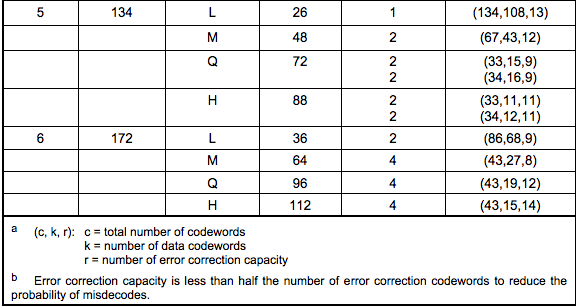# QR CODE Error correcting code

Above we have mentioned some error correction levels, Error Correction Code Level, there are four levels of error correction in the QR code, which is why the QR code is broken and can be swept out, which is why someone is in the center of the QR code. Add an icon.

Error correction capacity
L level7% of the code can be corrected
M level15% of the code can be corrected
Q level25% of the code can be corrected
H level30% of the code can be corrected

So, how does QR add error correction code to the data code? First, we need to group the data codes, that is, divide them into different blocks, and then perform error correction coding on each block. For how to group, we can view Table-13 to Table- on page 33 to page 44 of QR Code Spec . 22 definition table. Note the last two columns:
• Number of Error Code Correction Blocks : How many blocks to divide.
• Error Correction Code Per Blocks : The number of codes in each block, the number of so-called codes, that is, how many 8-bit bytes there are.For example: the above Version 5 + Q error correction level: requires 4 Blocks (2 blocks are a group, a total of two groups), 15 blocks of data in the first block of the two blocks + 9 bits each Error Correction Code (Note: The codewords in the table is an 8-bit byte) (Re-note: The formula for (c, k, r) in the last example is: c = k + 2 * r, because the footnote explained: The size of the error code is less than half of the error correction code)

The following figure gives a 5-Q example (because the binary will make the table too large, so I used decimal, we can see that each block of error correction code has 18 codewords, that is, 18 8-bit binary number)

groupPiecedataError correction code for each block
1167 85 70 134 87 38 85 194 119 50 6 18 6 103 38213 199 11 45 115 247 241 223 229 248 154 117 154 111 86 161 111 39
2246 246 66 7 118 134 242 7 38 86 22 198 199 146 687 204 96 60 202 182 124 157 200 134 27 129 209 17 163 163 120 133
21182 230 247 119 50 7 118 134 87 38 82 6 134 151 50 7148 116 177 212 76 133 75 242 238 76 195 230 189 10 108 240 192 141
270 247 118 86 194 6 151 50 16 236 17 236 17 236 17 236235 159 5 173 24 147 59 33 106 40 255 172 82 2 131 32 178 236
Note: The error correction code of the two-dimensional code is mainly realized by Reed-Solomon error correction (Reed-Solomon error correction algorithm). For this algorithm, it is quite complicated for me. There are many mathematical calculations, such as polynomial division, which maps the number of 1-255 to the nth power of 2 (0<=n<=255). The god-like things like the Galois Field, and the error-correcting mathematical formulas based on these foundations, because my data foundation is poor, it is too complicated for me, so I haven’t figured it out for a while. In the study, therefore, I am not here to say these things. Please also forgive me. (Of course, if a friend understands it, please consult me.)